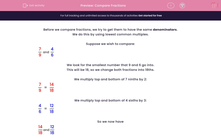# Compare Fractions

In this worksheet, students use common denominators to compare fractions.Key stage:  KS 2

Curriculum topic:   Maths and Numerical Reasoning

Curriculum subtopic:   Fractions

Difficulty level:#### Worksheet Overview

Before we compare fractions, we try to get them to have the same denominators.

We do this by using lowest common multiples.

Suppose we wish to compare:

 7 and 4 9 6

We look for the smallest number that 9 and 6 go into.

This will be 18, so we change both fractions into 18ths.

We multiply top and bottom of 7 ninths by 2:

 7 = 14 9 18

We multiply top and bottom of 4 sixths by 3:

 4 = 12 6 18

So we now have

 14 and 12 18 18

Clearly

 14 > 12 18 18

So

 7 > 4 9 6

Example

Which is bigger?

 12 or 11 8 6

The lowest common multiple of 8 and 6 is 24, because 3 × 8 = 24 and 4 × 6 = 24.

We multiply top and bottom of 12 eighths by 3:

 12 = 36 8 24

We multiply top and bottom of 11 sixths by 4:

 11 = 44 6 24

Clearly

 44 > 36 24 24

So

 11 is bigger 6

### What is EdPlace?

We're your National Curriculum aligned online education content provider helping each child succeed in English, maths and science from year 1 to GCSE. With an EdPlace account you’ll be able to track and measure progress, helping each child achieve their best. We build confidence and attainment by personalising each child’s learning at a level that suits them.

Get started••••